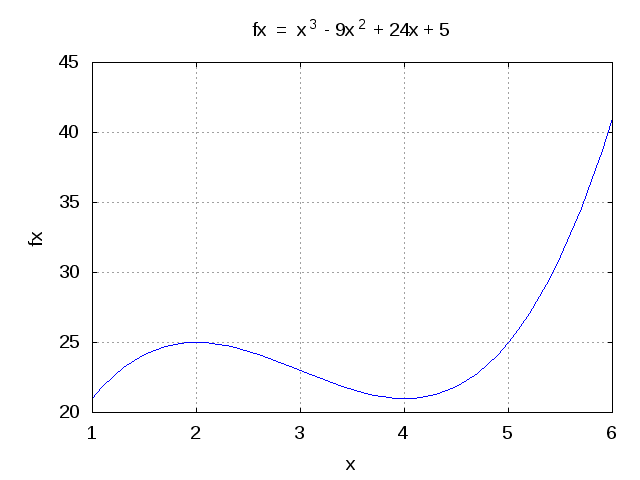- DSP log - http://www.dsplog.com -

GATE-2012 ECE Q46 (math)

Posted By Krishna Sankar On October 23, 2012 @ 6:44 am In GATE | 1 Comment

Question 46 on math from GATE (Graduate Aptitude Test in Engineering) 2012 Electronics and Communication Engineering paper.

Q46. The maximum value of  in the interval [1, 6] is

(A) 21

(B) 25

(C) 41

(D) 46Solution

Let us start by finding the critical points of the function .

The first derivative is,

.

Solving by setting .

.

Taking second derivative, .

At and this corresponds to a local minimum.

At and this corresponds to a local maximum.

The value of the function at these critical points are,

.

Hold on, we are not done yet…From the wiki entry on Extereme Value Theorem 

In calculus, the extreme value theorem states that if a real-valued function f is continuous in the closed and bounded interval [a,b], then f must attain its maximum and minimum value, each at least once. That is, there exist numbers c and d in [a,b] such that:

.

Further, from wiki entry on finding maxima and minima ,

If a function is continuous on a closed interval, then by the extreme value theorem  global maxima and minima exist. Furthermore, a global maximum (or minimum) either must be a local maximum (or minimum) in the interior of the domain, or must lie on the boundary of the domain. So a method of finding a global maximum (or minimum) is to look at all the local maxima (or minima) in the interior, and also look at the maxima (or minima) of the points on the boundary; and take the biggest (or smallest) one.

For our example, the function is real valued and is continuous in the closed and bounded interval [1,6].

Finding out the value of the function at the boundaries,

.

So the global maximum of the function  in the interval [1, 6] is  and it occurs at the boundary i.e. x = 6.

Matlab example

clear all; close all;
x = [1:.1:6];
fx = x.^3 - 9*x.^2 + 24*x + 5;
plot(x,fx);
xlabel('x'); ylabel('fx');
grid on; axis([1 6 20 45]);
title('fx  =  x^3 - 9x^2 + 24x + 5');Figure : Plot of the function  in the interval [1,6]

From the figure, it is relatively easy to observe that the maximum is hit at x=6 (and not at the local maximum point of x=2).

References

 GATE Examination Question Papers [Previous Years] from Indian Institute of Technology, Madras http://gate.iitm.ac.in/gateqps/2012/ec.pdf

 Wiki entry on Extreme Value Theorem

 Wiki entry on Maxima and Minima

URL to article: http://www.dsplog.com/2012/10/23/gate-2012-ece-q46-math/

URLs in this post:

 Extereme Value Theorem: http://en.wikipedia.org/wiki/Extreme_value_theorem

 wiki entry on finding maxima and minima: http://en.wikipedia.org/wiki/Maxima_and_minima#Finding_functional_maxima_and_minima

 http://en.wikipedia.org/wiki/Maxima_and_minima: http://en.wikipedia.org/wiki/Maxima_and_minima